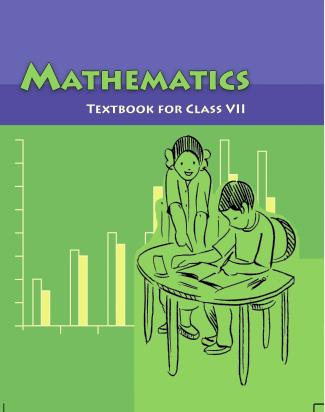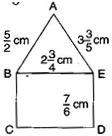1. /
2. CBSE
3. /
4. Class 07
5. /
6. Mathematics
7. /
8. NCERT Solutions for Class...

# NCERT Solutions for Class 7 Maths Exercise 2.1### myCBSEguide App

Download the app to get CBSE Sample Papers 2023-24, NCERT Solutions (Revised), Most Important Questions, Previous Year Question Bank, Mock Tests, and Detailed Notes.

NCERT solutions for Maths Fractions and Decimals## NCERT Solutions for Class 7 Maths Fractions and Decimals

###### Question 1.Solve:

(i){tex}2 – \frac{3}{5}{/tex}

(ii){tex}4 + \frac{7}{8}{/tex}

(iii){tex}\frac{3}{5} + \frac{2}{7}{/tex}

(iv){tex}\frac{9}{{11}} – \frac{4}{{15}}{/tex}

(v){tex}\frac{7}{{10}} + \frac{2}{5} + \frac{3}{2}{/tex}

(vi){tex}2\frac{2}{3} + 3\frac{1}{2}{/tex}

(vii){tex}8\frac{1}{2} – 3\frac{5}{8}{/tex}

(i) {tex}2 – \frac{3}{5}{/tex} = {tex}\frac{{10 – 3}}{5} = \frac{7}{5}{/tex} = {tex}1\frac{2}{5}{/tex}

(ii){tex}4 + \frac{7}{8}{/tex} = {tex}\frac{{32 + 7}}{8} = \frac{{39}}{8}{/tex} = {tex}4\frac{7}{8}{/tex}

(iii){tex}\frac{3}{5} + \frac{2}{7}{/tex} = {tex}\frac{{21 + 10}}{{35}}{/tex} = {tex}\frac{{31}}{{35}}{/tex}

(iv){tex}\frac{9}{{11}} – \frac{4}{{15}}{/tex} = {tex}\frac{{135 – 44}}{{165}} = \frac{{91}}{{165}}{/tex}

(v) {tex}\frac{7}{{10}} + \frac{2}{5} + \frac{3}{2}{/tex} = {tex}\frac{{7 + 4 + 15}}{{10}}{/tex} = {tex}\frac{{26}}{{10}} = \frac{{13}}{5}{/tex} = {tex}2\frac{3}{5}{/tex}

(vi) {tex}2\frac{2}{3} + 3\frac{1}{2}{/tex} = {tex}\frac{8}{3} + \frac{7}{2}{/tex} = {tex}\frac{{16 + 21}}{6}{/tex} = {tex}\frac{{37}}{6}{/tex} = {tex}6\frac{1}{6}{/tex}

(vii){tex}8\frac{1}{2} – 3\frac{5}{8}{/tex} = {tex}\frac{{17}}{2} – \frac{{29}}{8}{/tex} = {tex}\frac{{68 – 19}}{8}{/tex} = {tex}\frac{{39}}{8}{/tex} = {tex}4\frac{7}{8}{/tex}

NCERT Solutions for Class 7 Maths Exercise 2.1

###### Question 2.Arrange the following in descending order:

(i) {tex}\frac{2}{9},\frac{2}{3},\frac{8}{{21}}{/tex}

(ii) {tex}\frac{1}{5},\frac{3}{7},\frac{7}{{10}}{/tex}

(i) {tex}\frac{2}{9},\frac{2}{3},\frac{8}{{21}}{/tex} {tex} \Rightarrow {/tex}{tex}\frac{{14}}{{63}},\frac{{42}}{{63}},\frac{{24}}{{63}}{/tex} [Converting into like fractions]

{tex} \Rightarrow {/tex} {tex}\frac{{42}}{{63}} > \frac{{24}}{{63}} > \frac{{14}}{{63}}{/tex} [Arranging in descending order]

Therefore, {tex}\frac{2}{3} > \frac{8}{{21}} > \frac{2}{9}{/tex}

(ii) {tex}\frac{1}{5},\frac{3}{7},\frac{7}{{10}}{/tex} {tex} \Rightarrow {/tex}{tex}\frac{{14}}{{70}},\frac{{30}}{{70}},\frac{{49}}{{70}}{/tex} [Converting into like fractions]

{tex} \Rightarrow {/tex} {tex}\frac{{49}}{{70}} > \frac{{30}}{{70}} > \frac{{14}}{{70}}{/tex} [Arranging in descending order]

Therefore, {tex}\frac{7}{{10}} > \frac{3}{7} > \frac{1}{5}{/tex}

NCERT Solutions for Class 7 Maths Exercise 2.1

###### Question 3.In a “magic square”, the sum of the numbers in each row, in each column and along the diagonals is the same. Is this a magic square?
 {tex}\frac{4}{{11}}{/tex} {tex}\frac{9}{{11}}{/tex} {tex}\frac{2}{{11}}{/tex} {tex}\frac{3}{{11}}{/tex} {tex}\frac{5}{{11}}{/tex} {tex}\frac{7}{{11}}{/tex} {tex}\frac{8}{{11}}{/tex} {tex}\frac{1}{{11}}{/tex} {tex}\frac{6}{{11}}{/tex}

{tex}\left( {{\text{Along the first row }}\frac{4}{{11}} + \frac{9}{{11}} + \frac{2}{{11}} = \frac{{15}}{{11}}} \right){/tex}Sum of first row = {tex}\frac{4}{{11}} + \frac{9}{{11}} + \frac{2}{{11}} = \frac{{15}}{{11}}{/tex} [Given]

Sum of second row = {tex}\frac{3}{{11}} + \frac{5}{{11}} + \frac{7}{{11}} = \frac{{3 + 5 + 7}}{{11}} = \frac{{15}}{{11}}{/tex}

Sum of third row = {tex}\frac{8}{{11}} + \frac{1}{{11}} + \frac{6}{{11}} = \frac{{8 + 1 + 6}}{{11}} = \frac{{15}}{{11}}{/tex}

Sum of first column = {tex}\frac{4}{{11}} + \frac{3}{{11}} + \frac{8}{{11}} = \frac{{4 + 3 + 8}}{{11}} = \frac{{15}}{{11}}{/tex}

Sum of second column = {tex}\frac{9}{{11}} + \frac{5}{{11}} + \frac{1}{{11}} = \frac{{9 + 5 + 1}}{{11}} = \frac{{15}}{{11}}{/tex}

Sum of third column = {tex}\frac{2}{{11}} + \frac{7}{{11}} + \frac{6}{{11}} = \frac{{2 + 7 + 6}}{{11}} = \frac{{15}}{{11}}{/tex}

Sum of first diagonal (left to right) = {tex}\frac{4}{{11}} + \frac{5}{{11}} + \frac{6}{{11}} = \frac{{4 + 5 + 6}}{{11}} = \frac{{15}}{{11}}{/tex}

Sum of second diagonal (left to right) = {tex}\frac{2}{{11}} + \frac{5}{{11}} + \frac{8}{{11}} = \frac{{2 + 5 + 8}}{{11}} = \frac{{15}}{{11}}{/tex}

Since the sum of fractions in each row, in each column and along the diagonals are same, therefore it s a magic square.

NCERT Solutions for Class 7 Maths Exercise 2.1

###### Question 4.A rectangular sheet of paper is {tex}12\frac{1}{2}{/tex} cm long and {tex}10\frac{2}{3}{/tex} cm wide. Find its perimeter.

Given: The sheet of paper is in rectangular form.

Length of sheet = {tex}12\frac{1}{2}{/tex} cm and Breadth of sheet = {tex}10\frac{2}{3}{/tex} cm

Perimeter of rectangle = 2 (length + breadth)

= {tex}2\left( {12\frac{1}{2} + 10\frac{2}{3}} \right){/tex} = {tex}2\left( {\frac{{25}}{2} + \frac{{32}}{3}} \right){/tex}

= {tex}2\left( {\frac{{25 \times 3 + 32 \times 2}}{6}} \right){/tex} = {tex}2\left( {\frac{{75 + 64}}{6}} \right){/tex}

= {tex}2 \times \frac{{139}}{6}{/tex} = {tex}\frac{{139}}{3} = 46\frac{1}{3}{/tex} cm.

Thus, the perimeter of the rectangular sheet is {tex}46\frac{1}{3}{/tex} cm.

NCERT Solutions for Class 7 Maths Exercise 2.1

###### Question 5.Find the perimeter of (i) {tex}\Delta {/tex}ABE, (ii) the rectangle BCDE in this figure. Whose perimeter is greater?

(i) In {tex}\Delta {\text{ABE,}}{/tex} AB = {tex}\frac{5}{2}{/tex} cm, BE = {tex}2\frac{3}{4}{/tex} cm, AE = {tex}3\frac{3}{5}{/tex} cm

The perimeter of {tex}\Delta {\text{ABE}}{/tex} = AB + BE + AE

= {tex}\frac{5}{2} + 2\frac{3}{4} + 3\frac{3}{5}{/tex} = {tex}\frac{5}{2} + \frac{{11}}{4} + \frac{{18}}{5}{/tex}

= {tex}\frac{{50 + 55 + 72}}{{20}}{/tex} = {tex}\frac{{177}}{{20}}{/tex} = {tex}8\frac{{17}}{{20}}{/tex} cm

Thus, the perimeter of {tex}\Delta {\text{ABE}}{/tex}is {tex}8\frac{{17}}{{20}}{/tex} cm.

(ii) In rectangle BCDE, BE = {tex}2\frac{3}{4}{/tex} cm, ED = {tex}\frac{7}{6}{/tex} cm

Perimeter of rectangle = 2 (length + breadth)

= {tex}2\left( {2\frac{3}{4} + \frac{7}{6}} \right){/tex} = {tex}2\left( {\frac{{11}}{4} + \frac{7}{6}} \right){/tex}

= {tex}2\left( {\frac{{33 + 14}}{{12}}} \right){/tex} = {tex}\frac{{47}}{6}{/tex} = {tex}7\frac{5}{6}{/tex} cm

Thus, the perimeter of rectangle BCDE is {tex}7\frac{5}{6}{/tex} cm.

Comparing the perimeter of triangle and that of rectangle,

{tex}8\frac{{17}}{{20}}{/tex} cm >{tex}7\frac{5}{6}{/tex} cm

Therefore, the perimeter of triangle ABE is greater than that of rectangle BCDE.

NCERT Solutions for Class 7 Maths Exercise 2.1

###### Question 6. Salil wants to put a picture in a frame. The picture is {tex}7\frac{3}{5}{/tex} cm wide. To fit in the frame the picture cannot be more than {tex}7\frac{3}{{10}}{/tex} cm wide. How much should the picture be trimmed?

Given: The width of the picture = {tex}7\frac{3}{5}{/tex} cm and the width of picture frame = {tex}7\frac{3}{{10}}{/tex} cm

Therefore, the picture should be trimmed = {tex}7\frac{3}{5} – 7\frac{3}{{10}}{/tex} = {tex}\frac{{38}}{5} – \frac{{73}}{{10}}{/tex}

= {tex}\frac{{76 – 73}}{{10}}{/tex} = {tex}\frac{3}{{10}}{/tex} cm

Thus, the picture should be trimmed by {tex}\frac{3}{{10}}{/tex} cm.

NCERT Solutions for Class 7 Maths Exercise 2.1

###### Question 7.Ritu ate {tex}\frac{3}{5}{/tex} part of an apple and the remaining apple was eaten by her brother Somu. How much part of the apple did Somu eat? Who had the larger share? By how much?

The part of an apple eaten by Ritu = {tex}\frac{3}{5}{/tex}

The part of an apple eaten by Somu = {tex}1 – \frac{3}{5} = \frac{{5 – 3}}{5} = \frac{2}{5}{/tex}

Comparing the parts of apple eaten by both Ritu and Somu {tex}\frac{3}{5} > \frac{2}{5}{/tex}

Larger share will be more by {tex}\frac{3}{5} – \frac{2}{5} = \frac{1}{5}{/tex} part.

Thus, Ritu’s part is {tex}\frac{1}{5}{/tex} more than Somu’s part.

NCERT Solutions for Class 7 Maths Exercise 2.1

###### Question 8.Michael finished colouring a picture in {tex}\frac{7}{{12}}{/tex} hour. Vaibhav finished colouring the same picture in {tex}\frac{3}{4}{/tex} hour. Who worked longer? By what fraction was it longer?

Time taken by Michael to colour the picture = {tex}\frac{7}{{12}}{/tex} hour

Time taken by Vaibhav to colour the picture = {tex}\frac{3}{4}{/tex} hour

Converting both fractions in like fractions, {tex}\frac{7}{{12}}{/tex} and {tex}\frac{{3 \times 3}}{{4 \times 3}} = \frac{9}{{12}}{/tex}

Here, {tex}\frac{7}{{12}}{/tex}<{tex}\frac{9}{{12}}{/tex} {tex} \Rightarrow {/tex}{tex}\frac{7}{{12}}{/tex}<{tex}\frac{3}{4}{/tex}

Thus, Vaibhav worked longer time.

Vaibhav worked longer time by {tex}\frac{3}{4} – \frac{7}{{12}} = \frac{{9 – 7}}{{12}} = \frac{2}{{12}} = \frac{1}{6}{/tex} hour.

Thus, Vaibhav took {tex}\frac{1}{6}{/tex} hour more than Michael.

## NCERT Solutions for Class 7 Maths Exercise 2.1

NCERT Solutions Class 7 Maths PDF (Download) Free from myCBSEguide app and myCBSEguide website. Ncert solution class 7 Maths includes text book solutions from Class 7 Maths Book . NCERT Solutions for CBSE Class 7 Maths have total 15 chapters. 7 Maths NCERT Solutions in PDF for free Download on our website. Ncert Maths class 7 solutions PDF and Maths ncert class 7 PDF solutions with latest modifications and as per the latest CBSE syllabus are only available in myCBSEguide.

## CBSE app for Students

To download NCERT Solutions for Class 7 Maths, Social Science Computer Science, Home Science, Hindi English, Maths Science do check myCBSEguide app or website. myCBSEguide provides sample papers with solution, test papers for chapter-wise practice, NCERT solutions, NCERT Exemplar solutions, quick revision notes for ready reference, CBSE guess papers and CBSE important question papers. Sample Paper all are made available through the best app for CBSE students and myCBSEguide website.Test Generator

Create question paper PDF and online tests with your own name & logo in minutes.myCBSEguide

Question Bank, Mock Tests, Exam Papers, NCERT Solutions, Sample Papers, Notes

### 2 thoughts on “NCERT Solutions for Class 7 Maths Exercise 2.1”

1. It is very helpful for me. I like it but there is not one question is available. So there have problem to me.

2. Thanks for this it is helpful for us